# 利用指标实时优化智能交易系统

22 十月 2018, 07:58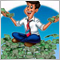0
3 291

### 1. 思路

1. 初始化测试指标时，历史记录按 М1 OHLC 价格计算。 在计算订单盈利/亏损时，首先检查止损，随后按最高价/最低价（取决于订单类型）检查止盈。
2. 根据第 1 点，订单仅在蜡烛开盘时下单。
3. 要减少测试指标的运行总数，请采用有意义的方法来选择其中使用的参数。 您可以在此处根据指标逻辑添加最小步幅和过滤参数。 例如，在使用 MACD 时，如果快速和慢速均线的参数范围重叠，则测试指标排除某一组参数重叠部分的运行，如慢速均线周期小于或等于快速均线周期的部分，这与 EA 操作逻辑相悖。 您还可以在区间内添加最小差值，初期丢弃大量假信号的选项。

### 2. 交易策略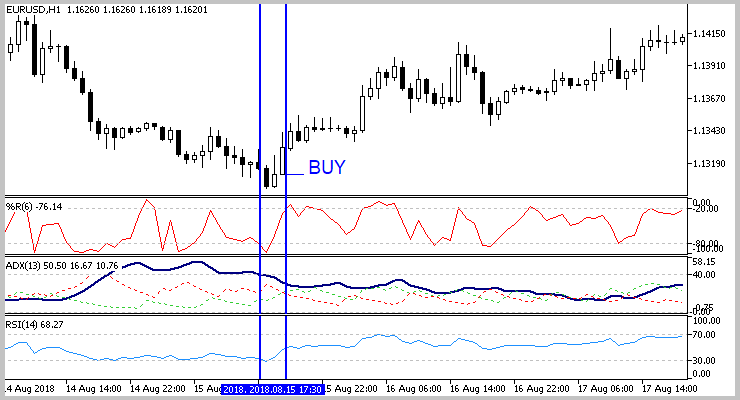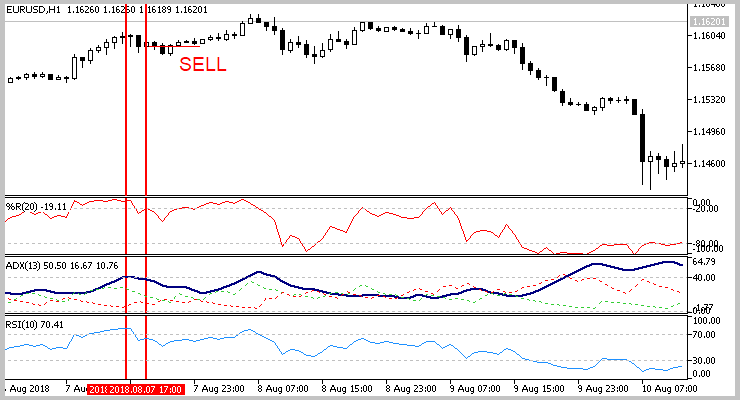### 3. 准备测试器指标

#### 3.1. 虚拟交易类

```bool CDeal::Tick(double price, int spread)
{
if(d_ClosePrice>0)
return true;
//---
switch(e_Direct)
{
if(d_SL_Price>0 && d_SL_Price>=price)
{
d_ClosePrice=price;
i_Profit=(int)((d_ClosePrice-d_OpenPrice)/d_Point);
}
else
{
if(d_TP_Price>0 && d_TP_Price<=price)
{
d_ClosePrice=price;
i_Profit=(int)((d_ClosePrice-d_OpenPrice)/d_Point);
}
}
break;
case POSITION_TYPE_SELL:
if(d_SL_Price>0 && d_SL_Price<=price)
{
d_ClosePrice=price;
i_Profit=(int)((d_OpenPrice-d_ClosePrice)/d_Point);
}
else
{
if(d_TP_Price>0 && d_TP_Price>=price)
{
d_ClosePrice=price;
i_Profit=(int)((d_OpenPrice-d_ClosePrice)/d_Point);
}
}
break;
}
return IsClosed();
}
```

#### 3.2. 指标编程

```input int                  HistoryDepth      =  500;           //历史数据深度(柱线)
input int                  StopLoss          =  200;           //止损(点数)
input int                  TakeProfit        =  600;           //止盈(点数)
//--- RSI 指标参数
input int                  RSIPeriod         =  28;            //RSI 周期
input double               RSITradeZone      =  30;            //超买/超卖区域大小
//--- WPR 指标参数
input int                  WPRPeriod         =  7;             // WPR 周期
input double               WPRTradeZone      =  30;            //超买/超卖区域大小
//---
input int                  Direction         =  -1;            //交易方向 "-1"-所有, "0"-买入, "1"-卖出
//---
input int                  AveragePeriod     =  10;            //均化周期
```

1. 盈利成交的概率。

`double      Buffer_Probability[];`

2. 测试期间的盈利因子。

`double      Buffer_ProfitFactor[];`

3. 止损和止盈等级。 可以通过创建匹配指标句柄的数组，并在 EA 中指定等级，或者在执行成交时通过其句柄请求指标参数来排除这两个缓冲区。 但是，目前的解决方案对我来说似乎是最简单的解决方案。

```double      Buffer_TakeProfit[];
double      Buffer_StopLoss[];
```

4. 用于计算测试区间内执行的成交总数及其盈利数量的缓冲区。

```double      Buffer_ProfitCount[];
double      Buffer_DealsCount[];
```

5. 以下两个缓冲区用于计算先前的数值，并且仅包含当前柱线的类似数据。

```double      Buffer_ProfitCountCurrent[];
double      Buffer_DealsCountCurrent[];
```

6. 最后但并不仅限于，缓冲区向 EA 发送信号以便执行成交。

`double      Buffer_TradeSignal[];`

```CArrayObj   Deals;
datetime    last_deal;
```

```int OnInit()
{
//--- 获取 RSI 指标句柄
rsi_handle=iRSI(Symbol(),PERIOD_CURRENT,RSIPeriod,PRICE_CLOSE);
if(rsi_handle==INVALID_HANDLE)
{
Print("Test Indicator",": Failed to get RSI handle");
Print("Handle = ",rsi_handle,"  error = ",GetLastError());
return(INIT_FAILED);
}
//--- 获取 WPR 指标句柄
wpr_handle=iWPR(Symbol(),PERIOD_CURRENT,WPRPeriod);

if(wpr_handle==INVALID_HANDLE)
{
Print("Test Indicator",": Failed to get WPR handle");
Print("Handle = ",wpr_handle,"  error = ",GetLastError());
return(INIT_FAILED);
}
{
Print("Test Indicator",": Failed to get ADX handle");
Print("Handle = ",adx_handle,"  error = ",GetLastError());
return(INIT_FAILED);
}
```

```//--- 指标缓存区映射
SetIndexBuffer(0,Buffer_Probability,INDICATOR_CALCULATIONS);
SetIndexBuffer(1,Buffer_DealsCount,INDICATOR_CALCULATIONS);
SetIndexBuffer(3,Buffer_ProfitFactor,INDICATOR_CALCULATIONS);
SetIndexBuffer(4,Buffer_ProfitCount,INDICATOR_CALCULATIONS);
SetIndexBuffer(5,Buffer_TakeProfit,INDICATOR_CALCULATIONS);
SetIndexBuffer(6,Buffer_StopLoss,INDICATOR_CALCULATIONS);
SetIndexBuffer(7,Buffer_DealsCountCurrent,INDICATOR_CALCULATIONS);
SetIndexBuffer(8,Buffer_ProfitCountCurrent,INDICATOR_CALCULATIONS);
```

```   ArraySetAsSeries(Buffer_Probability,true);
ArraySetAsSeries(Buffer_ProfitFactor,true);
ArraySetAsSeries(Buffer_DealsCount,true);
ArraySetAsSeries(Buffer_ProfitCount,true);
ArraySetAsSeries(Buffer_TakeProfit,true);
ArraySetAsSeries(Buffer_StopLoss,true);
ArraySetAsSeries(Buffer_DealsCountCurrent,true);
ArraySetAsSeries(Buffer_ProfitCountCurrent,true);
//---
ArraySetAsSeries(rsi,true);
ArraySetAsSeries(wpr,true);
```

```   Deals.Clear();
last_deal=0;
//---
IndicatorSetString(INDICATOR_SHORTNAME,"Test Indicator");
//---
return(INIT_SUCCEEDED);
}
```

```int GetIndValue(int depth)
{
return -1;
//---
return depth;
}
```

1. 重新计算多根柱线时（初始化后第一次启动，或一根新柱线）。 在此流程中，我们将根据 M1 时间帧的历史数据在每根未计算的柱线上检查入场信号，并处理成交持仓的止损单。
2. 在尚未形成新柱时，在每次逐笔报价上检查持仓的止损单的激活情况。

```int OnCalculate(const int rates_total,
const int prev_calculated,
const datetime &time[],
const double &open[],
const double &high[],
const double &low[],
const double &close[],
const long &tick_volume[],
const long &volume[],
{
//---
int total=rates_total-prev_calculated;
if(prev_calculated<=0)
{
total=fmin(total,HistoryDepth);
//---
ArrayInitialize(Buffer_Probability,0);
ArrayInitialize(Buffer_ProfitFactor,0);
ArrayInitialize(Buffer_DealsCount,0);
ArrayInitialize(Buffer_ProfitCount,0);
ArrayInitialize(Buffer_TakeProfit,TakeProfit*_Point);
ArrayInitialize(Buffer_StopLoss,StopLoss*_Point);
ArrayInitialize(Buffer_DealsCountCurrent,0);
ArrayInitialize(Buffer_ProfitCountCurrent,0);
}
```

```   if(total>0)
{
total=MathMin(GetIndValue(total+2),rates_total);
if(total<=0)
return prev_calculated;
```

```      if(!ArraySetAsSeries(open,true) || !ArraySetAsSeries(high,true) || !ArraySetAsSeries(low,true) || !ArraySetAsSeries(close,true)
return prev_calculated;
```

```      for(int i=total-3;i>=0;i--)
{
Buffer_TakeProfit[i]=TakeProfit*_Point;
Buffer_StopLoss[i]=StopLoss*_Point;
Buffer_DealsCount[i]=Buffer_DealsCountCurrent[i]=0;
Buffer_ProfitCount[i]=Buffer_ProfitCountCurrent[i]=0;
```

```         if(last_deal<time[i])
{
{
double sl=open_price-StopLoss*_Point;
double tp=open_price+TakeProfit*_Point;
if(temp!=NULL)
}
if(SellSignal(i))
{
double open_price=open[i];
double sl=open_price+StopLoss*_Point;
double tp=open_price-TakeProfit*_Point;
CDeal *temp=new CDeal(_Symbol,rates_total-i,POSITION_TYPE_SELL,time[i],open_price,sl,tp);
if(temp!=NULL)
}
else /*SellSignal*/
}
```

```         if(Deals.Total()>0)
{
if(PeriodSeconds()!=60)
{
MqlRates rates[];
int rat=CopyRates(_Symbol,PERIOD_M1,time[i],(i>0 ? time[i-1] : TimeCurrent()),rates);
```

```               int closed=0, profit=0;
for(int r=0;(r<rat && Deals.Total()>0);r++)
{
if(closed>0)
{
Buffer_DealsCountCurrent[i]+=closed;
Buffer_ProfitCountCurrent[i]+=profit;
}
}
```

```               if(rat<0)
{
Buffer_DealsCountCurrent[i]+=closed;
Buffer_ProfitCountCurrent[i]+=profit;
}
}
else /* PeriodSeconds()!=60 */
{
int closed=0, profit=0;
Buffer_DealsCountCurrent[i]+=closed;
Buffer_ProfitCountCurrent[i]+=profit;
}
} /* Deals.Total()>0 */
```

```         Buffer_DealsCount[i+1]=NormalizeDouble(Buffer_DealsCount[i+2]+Buffer_DealsCountCurrent[i+1]-((i+HistoryDepth+1)<rates_total ? Buffer_DealsCountCurrent[i+HistoryDepth+1] : 0),0);
Buffer_ProfitCount[i+1]=NormalizeDouble(Buffer_ProfitCount[i+2]+Buffer_ProfitCountCurrent[i+1]-((i+HistoryDepth+1)<rates_total ? Buffer_ProfitCountCurrent[i+HistoryDepth+1] : 0),0);
Buffer_DealsCount[i]=NormalizeDouble(Buffer_DealsCount[i+1]+Buffer_DealsCountCurrent[i]-((i+HistoryDepth)<rates_total ? Buffer_DealsCountCurrent[i+HistoryDepth] : 0),0);
Buffer_ProfitCount[i]=NormalizeDouble(Buffer_ProfitCount[i+1]+Buffer_ProfitCountCurrent[i]-((i+HistoryDepth)<rates_total ? Buffer_ProfitCountCurrent[i+HistoryDepth] : 0),0);
```

```         if(Buffer_DealsCount[i]>0)
{
double pr=2.0/(AveragePeriod-1.0);
Buffer_Probability[i]=((i+1)<rates_total && Buffer_Probability[i+1]>0 && Buffer_DealsCount[i+1]>=AveragePeriod ? Buffer_ProfitCount[i]/Buffer_DealsCount[i]*100*pr+Buffer_Probability[i+1]*(1-pr) : Buffer_ProfitCount[i]/Buffer_DealsCount[i]*100);
if(Buffer_DealsCount[i]>Buffer_ProfitCount[i])
{
double temp=(Buffer_ProfitCount[i]*TakeProfit)/(StopLoss*(Buffer_DealsCount[i]-Buffer_ProfitCount[i]));
Buffer_ProfitFactor[i]=((i+1)<rates_total && Buffer_ProfitFactor[i+1]>0 ? temp*pr+Buffer_ProfitFactor[i+1]*(1-pr) : temp);
}
else
Buffer_ProfitFactor[i]=TakeProfit*Buffer_ProfitCount[i];
}
}
}
```

```bool CheckDeals(double open,double high,double low,double close,int spread,datetime time,int &closed, int &profit)
{
closed=0;
profit=0;
bool result=true;
```

```   for(int i=0;i<Deals.Total();i++)
{
CDeal *deal=Deals.At(i);
if(CheckPointer(deal)==POINTER_INVALID)
{
if(Deals.Delete(i))
i--;
else
result=false;
continue;
}
```

```      if(deal.GetTime()>time)
continue;
```

```      if(deal.Tick(open,spread))
{
closed++;
if(deal.GetProfit()>0)
profit++;
if(Deals.Delete(i))
i--;
if(CheckPointer(deal)!=POINTER_INVALID)
delete deal;
continue;
}
switch(deal.Type())
{
{
closed++;
if(deal.GetProfit()>0)
profit++;
if(Deals.Delete(i))
i--;
if(CheckPointer(deal)!=POINTER_INVALID)
delete deal;
continue;
}
{
closed++;
if(deal.GetProfit()>0)
profit++;
if(Deals.Delete(i))
i--;
if(CheckPointer(deal)!=POINTER_INVALID)
delete deal;
continue;
}
break;
case POSITION_TYPE_SELL:
{
closed++;
if(deal.GetProfit()>0)
profit++;
if(Deals.Delete(i))
i--;
if(CheckPointer(deal)!=POINTER_INVALID)
delete deal;
continue;
}
{
closed++;
if(deal.GetProfit()>0)
profit++;
if(Deals.Delete(i))
i--;
if(CheckPointer(deal)!=POINTER_INVALID)
delete deal;
continue;
}
break;
}
}
//---
return result;
}
```

### 4. 创建 EA

```input double               Lot                     =  0.01;
input int                  HistoryDepth            =  500;           //历史数据深度(柱线)
//--- RSI 指标参数
input int                  RSIPeriod_Start         =  5;             //RSI 周期
input int                  RSIPeriod_Stop          =  30;            //RSI 周期
input int                  RSIPeriod_Step          =  5;             //RSI 周期
//---
input double               RSITradeZone_Start      =  30;            //起始超买/超卖区域大小
input double               RSITradeZone_Stop       =  30;            //结束超买/超卖区域大小
input double               RSITradeZone_Step       =  5;             //超买/超卖区域大小步幅
//--- WPR 指标参数
input int                  WPRPeriod_Start         =  5;             //WPR 起始周期
input int                  WPRPeriod_Stop          =  30;            //WPR 结束周期
input int                  WPRPeriod_Step          =  5;             //WPR 周期步幅
//---
input double               WPRTradeZone_Start      =  20;            //超买/超卖区域大小起始
input double               WPRTradeZone_Stop       =  20;            //超买/超卖区域大小结束
input double               WPRTradeZone_Step       =  5;             //超买/超卖区域大小步幅
//---
//--- 成交设置
input int                  TakeProfit_Start        =  600;           //止盈起始
input int                  TakeProfit_Stop         =  600;           //止盈结束
input int                  TakeProfit_Step         =  100;           //止盈步幅
//---
input int                  StopLoss_Start          =  200;           //止损起始
input int                  StopLoss_Stop           =  200;           //止损结束
input int                  StopLoss_Step           =  100;           //止损步幅
//---
input double               MinProbability          =  60.0;          //Minimal Probability
input double               MinProfitFactor         =  1.6;           //Minimal Profitfactor
input int                  MinOrders               =  10;            //历史当中最少成交数量
```

```CArrayInt   ar_Handles;
```

```int OnInit()
{
//---
for(int rsi=RSIPeriod_Start;rsi<=RSIPeriod_Stop;rsi+=RSIPeriod_Step)
for(int wpr=WPRPeriod_Start;wpr<=WPRPeriod_Stop;wpr+=WPRPeriod_Step)
for(int tp=TakeProfit_Start;tp<=TakeProfit_Stop;tp+=TakeProfit_Step)
for(int sl=StopLoss_Start;sl<=StopLoss_Stop;sl+=StopLoss_Step)
for(int dir=0;dir<2;dir++)
{
int handle=iCustom(_Symbol,PERIOD_CURRENT,"::Indicators\\TestIndicator\\TestIndicator.ex5",HistoryDepth,
sl,
tp,
rsi,
rsi_tz,
wpr,
wpr_tz,
dir);
if(handle==INVALID_HANDLE)
return INIT_FAILED;
}
```

```   Trade.SetAsyncMode(false);
return INIT_FAILED;
//---
return(INIT_SUCCEEDED);
}
```

```void OnTick()
{
//---
static datetime last_bar=0;
datetime cur_bar=(datetime)SeriesInfoInteger(_Symbol,PERIOD_CURRENT,SERIES_LASTBAR_DATE);
if(cur_bar==last_bar)
return;
```

```   if(PositionSelect(_Symbol))
{
last_bar=cur_bar;
return;
}
```

```   int signal=0;
double probability=0;
double profit_factor=0;
double tp=0,sl=0;
bool ind_caclulated=false;
double temp[];
for(int i=0;i<ar_Handles.Total();i++)
{
if(CopyBuffer(ar_Handles.At(i),2,1,1,temp)<=0)
continue;
ind_caclulated=true;
if(temp==0)
continue;
```

```      if(signal!=0 && temp!=signal)
{
last_bar=cur_bar;
return;
}
signal=(int)temp;
```

```      if(CopyBuffer(ar_Handles.At(i),1,1,1,temp)<=0 || temp<MinOrders)
continue;
```

```      if(CopyBuffer(ar_Handles.At(i),0,1,1,temp)<=0 || temp<MathMax(probability,MinProbability))
continue;
```

```      if(MathAbs(temp-probability)<=1)
{
double ind_probability=temp;
//---
if(CopyBuffer(ar_Handles.At(i),3,1,1,temp)<=0 || temp<MathMax(profit_factor,MinProfitFactor))
continue;
double ind_profit_factor=temp;
if(CopyBuffer(ar_Handles.At(i),5,1,1,temp)<=0)
continue;
double ind_tp=temp;
if(CopyBuffer(ar_Handles.At(i),6,1,1,temp)<=0)
continue;
double ind_sl=temp;
if(MathAbs(ind_profit_factor-profit_factor)<=0.01)
{
if(sl<=0 || tp/sl>=ind_tp/ind_sl)
continue;
}
//---
probability=ind_probability;
profit_factor=ind_profit_factor;
tp=ind_tp;
sl=ind_sl;
}
```

```      else /* MathAbs(temp-probability)<=1 */
{
double ind_probability=temp;
//---
if(CopyBuffer(ar_Handles.At(i),3,1,1,temp)<=0 || temp<MinProfitFactor)
continue;
double ind_profit_factor=temp;
if(CopyBuffer(ar_Handles.At(i),5,1,1,temp)<=0)
continue;
double ind_tp=temp;
if(CopyBuffer(ar_Handles.At(i),6,1,1,temp)<=0)
continue;
double ind_sl=temp;
probability=ind_probability;
profit_factor=ind_profit_factor;
tp=ind_tp;
sl=ind_sl;
}
}
```

```   if(!ind_caclulated)
return;
```

```   last_bar=cur_bar;
//---
if(signal==0 || probability==0 || profit_factor==0 || tp<=0 || sl<=0)
return;
```

```   if(signal==1)
{
tp+=price;
sl=price-sl;
}
else
if(signal==-1)
{
double price=SymbolInfoDouble(_Symbol,SYMBOL_BID);
tp=price-tp;
sl+=price;
}
}
```

### 5. 测试方法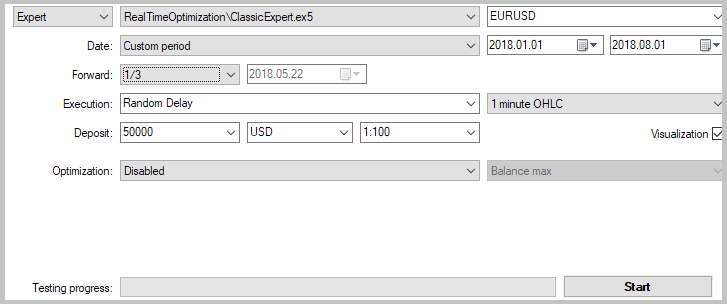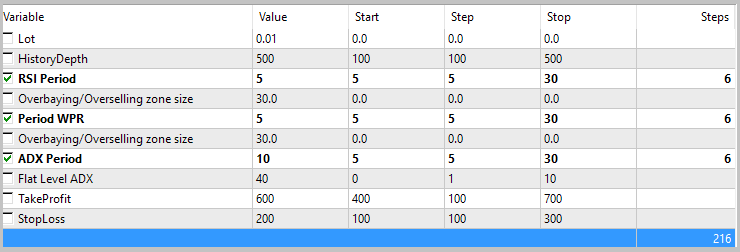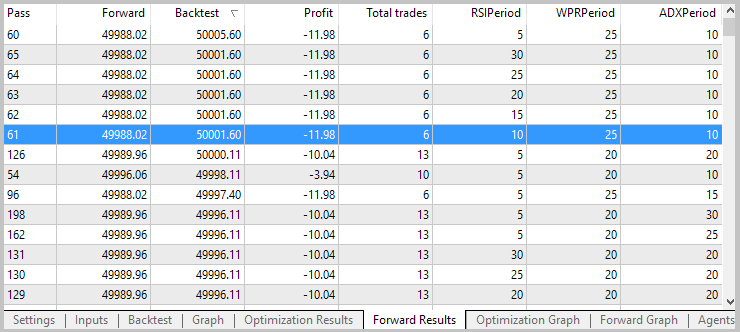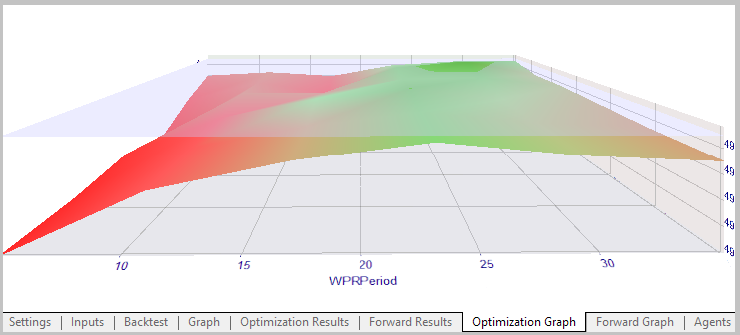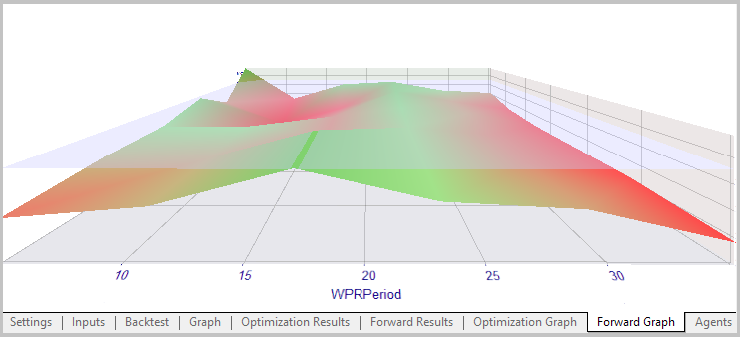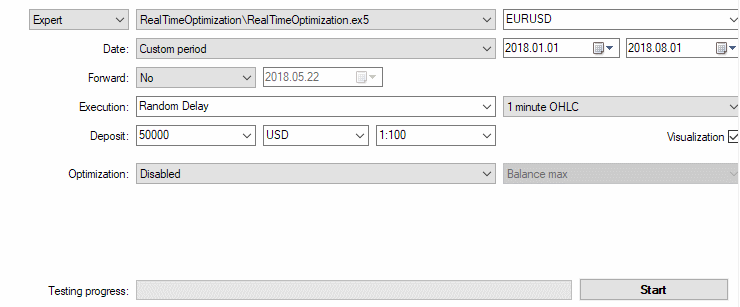### 本文中使用的程序

#

1 Deal.mqh 类库 虚拟交易类
2 TestIndicator.mq5 指标 测试指标
3 RealTimeOptimization.mq5 智能交易系统 基于提议方法的 EA
4 ClassicExpert.mq5 智能交易系统 基于标准方法的 EA，用于优化比较

MQL5.zip (360.42 KB)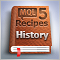MQL5 酷客宝典: 读取持有锁仓仓位的属性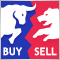Elder-Ray (多头力度和空头力度)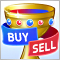反向交易: 圣杯还是危险的假象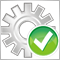自动优化 MetaTrader 5 专用 EA